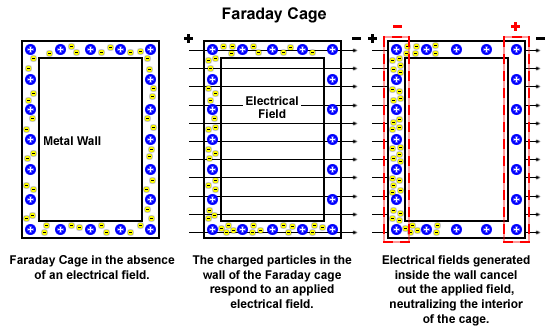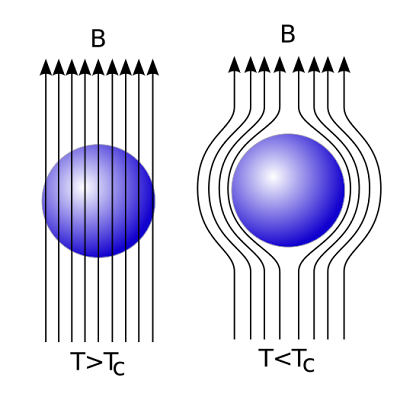## Tuesday, April 2, 2013

### Is Gravity Control Propulsion viable? Part 3

If one does a survey of the online preprints on arXiv and viXra and does a search for terms gravity and quantum vacuum, one will find a large number of papers dealing with the subject. Most of the papers that I went through appear to be highly speculative or introduce many assumptions to their models or have contradictions to known Physics. The papers that were more useful described their model rigorously and propose an experiment to verify their hypothesis with testable predictions together with good references.

Getting back to assessing the viability of gravity control propulsion, our inability to understand the physical mechanism of the bending of spacetime by mass and energy density is the main stumbling block to answering this question. All current proposed alternative models to GR are either unconfirmed, speculative or have their own problems.

Let's have a look at the following paper by Alfonso Rueda and Bernard Haisch on Gravity and the Quantum Vacuum Inertia Hypothesis. We'll first note "Neither our approach nor the conventional presentations of GR for that matter, can offer a physical explanation of the mechanism of the bending of spacetime as related to energy density", however from their hypothesis the authors explain:

"Inertial mass arises upon acceleration through the electromagnetic quantum vacuum, whereas gravitational mass — as manifest in weight — results from what may in a limited sense be viewed as acceleration of the electromagnetic quantum vacuum past a fixed object. The latter case occurs when an object is held fixed in a gravitational field and the quantum vacuum radiation associated with the freely-falling frame instantaneously comoving with the object follows curved geodesics as prescribed by general relativity."
In others words one only feels weight while for eg sitting on a chair but not while in free fall when jumping out of an airplane. We know the quantum vacuum consists of a sea of particles created and annihilated in very short time frames (these are called virtual particles). Among these virtual pairs of particles are positive and negative electrons popping in and out of existence. Note that this process is also responsible for determining the universal speed limit of light, mass and energy in vacuum  $c_{0}=\frac{1}{\sqrt{\mu_{0}\epsilon_{0}}}$  (see The quantum vacuum as the origin of the speed of light). Is an accelerating quantum vacuum with its sea of virtual particles interacting with every atom in an object and responsible for weight? Is gravity electromagnetic in origin? If this were the case one still has to explain the physics of what causes the acceleration of the quantum vacuum by mass and energy density. According to the paper the Quantum Vacuum Inertia Hypothesis is consistent with GR and the authors derive the same result using both SED and QED. They also propose that "at least part of the inertial force of opposition to acceleration, or inertia reaction force, springs from the electromagnetic quantum vacuum." This Rindler frame force as it is called stems from the local interaction of mass with the electromagnetic quantum vacuum when accelerated however there is no force on objects with constant velocity (inertial frames) and is proportional to acceleration imparted on the object.
"We have called the notion that at least part of the inertia of an object should be due to the individual and collective interaction of its quarks and electrons with the quantum vacuum as the quantum vacuum inertia hypothesis with the proviso that analogous contributions are expected from the other bosonic vacuum fields."
The Rindler frame force (or the vacuum electromagnetic counteracting reaction force) is derived as
$-f^{zp}=\left[\frac{V_{0}}{c^{2}}\int\eta\left(\omega\right)\rho\left(\omega\right)d\omega\right]a.$ This is a description of the electromagnetic component of the proposed reaction force and it remains to be seen what the other components of the force are. An experiment is proposed with a cavity resonator to verify the following: "First, some confirmation would be given to the electromagnetic vacuum contribution to inertial mass concept, or quantum vacuum inertia hypothesis. An important confirmation would be the reality — or virtuality — of the zero-point field."

Note that if the above hypothesis is confirmed, this would rule out Mach's principle and inertia would be shown to be purely a local effect between mass and the quantum vacuum (I tend towards the local effect hypothesis and think Mach's principle is incorrect). The paper also suggests that gravity even in part is electromagnetic in origin so before continuing on let us look at what options we have for electromagnetic shielding.

In Part 1 we looked at the hypothetical gravitational shielding concept. Although unrelated, it is useful to explore the following two effects in physics which are confirmed by experiment. Electromagnetic shielding or the Faraday cage is widely used in electronics and the lab to exclude both static and non-static electric fields inside a metal cage which is due to the electrons in the metal shield rearranging themselves to effectively cancel the outside electric field inside the cage as shown below. It will also shield the inside from the fluctuating magnetic field component of electromagnetic waves (such as radio waves) but cannot block static or slowly varying magnetic fields (such as the Earth's magnetic field).

﻿The Faraday cage excludes electric fields inside the cage. Image: Magnet Lab

For magnetic fields we have the Meissner effect, a property of superconductors which exclude magnetic fields inside the object when a critical cold temperature is reached as shown below and this also applies to hollow superconductor objects.The Meissner effect for superconductors, magnetic field lines are excluded from the object when a critical cold temperature is reached. Image: Wiki

Questions:

• If the dynamic quantum vacuum is partly responsible for gravitational effects as suggested in the paper, is there a means to shield an object from the accelerating quantum vacuum?
• Can we stop the interaction of electrons and quarks of the object inside the shield from the outside accelerating dynamic quantum vacuum?
• If a shielded object was disconnected from the outside quantum vacuum, how would this affect the object's mass and the quantum vacuum inside?
• Is it plausible to have a static quantum vacuum within an accelerating quantum vacuum?

Getting back to the paper the Equivalence Principle is explained:

"The physical basis for the principle of equivalence is the fact that accelerating through the electromagnetic quantum vacuum is identical to remaining fixed in a gravitational field and having the electromagnetic quantum vacuum fall past on curved geodesics."
According to the paper the hypothesis appears to agree with GR and also explains the bending of light near mass etc "However this does not mean that we have explained the mechanism for the actual bending of space-time in the vicinity of a material object. This is the origin of so-called active gravitational mass that still requires an explanation within the viewpoint of the quantum vacuum inertia hypothesis." There's a useful reference to the (highly recommended) textbook Gravitation by  Misner, Thorne and Wheeler and points to Chapter 17 "How mass-energy generates curvature" which describes the alternative models and provides a good insight on the various approaches to this problem. The sixth model by Sakharov begins with general vacuum considerations as did the authors of this paper.

The paper also gives an explanation of mass "it was made clear that within the quantum vacuum inertia hypothesis proposed therein, the mass of the object, m, could be viewed as the energy in the equivalent electromagnetic quantum vacuum field captured within the structure of the object and that readily interacts with the object."

So it appears from the above hypothesis that the key is to understand how electrons and quarks in  atoms interact with the dynamic quantum vacuum. Note that as the authors point out though we also haven't established the mechanism responsible for the acceleration of the quantum vacuum due to mass and energy density. Getting back to the original question regarding Gravity Control Propulsion it appears that this answer cannot also be answered by GR, the current alternate models to GR or the above hypothesis, however they do provide some good pointers to follow. The problem is pointing us to quantum physics and particle physics, we lack the understanding of the quantum processes that occur between matter - dynamic quantum vacuum interactions. The particles mentioned in the paper ie electrons and quarks both have electric charge. We cannot interact with quarks as they are locked in the nucleus of protons and neutrons due to colour confinement so we will look at the lepton family of particles. We'll continue with this next time. Till then ponder on the Koide formula below:

$Q=\frac{m_{e}+m_{\mu}+m_{\tau}}{\left(\sqrt{m_{e}}+\sqrt{m_{\mu}}+\sqrt{m_{\tau}}\right)^{2}}\thickapprox\frac{2}{3}$

CI.